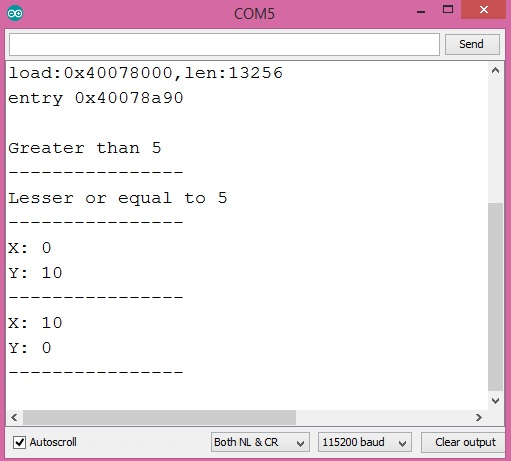# 二十四，ESP32 条件运算符

## 一，介绍

```Expression1 ? Expression2 : Expression3
```

• ESP32开发板

## 四，代码设计

```Serial.begin(115200);
```

```int cond = 10;
```

```String result = cond > 5 ? "Greater than 5" : "Lesser or equal to 5";
```

```cond = 3;

result = cond > 5 ? "Greater than 5" : "Lesser or equal to 5";
Serial.println(result);
```

```bool condition = false;
```

```int x = 0, y = 0;
```

```(condition ? x : y) = 10;

Serial.println(x);
Serial.println(y);
```

```condition = true;
x=0;
y=0;

(condition ? x : y) = 10;

Serial.println(x);
Serial.println(y);
```

```void setup() {

Serial.begin(115200);
Serial.println();

int cond = 10;

String result = cond > 5 ? "Greater than 5" : "Lesser or equal to 5";
Serial.println(result);
Serial.println("----------------");

cond = 3;

result = cond > 5 ? "Greater than 5" : "Lesser or equal to 5";
Serial.println(result);
Serial.println("----------------");

bool condition = false;
int x = 0, y = 0;

(condition ? x : y) = 10;

Serial.print("X: ");
Serial.println(x);
Serial.print("Y: ");
Serial.println(y);
Serial.println("----------------");

condition = true;
x=0;
y=0;

(condition ? x : y) = 10;

Serial.print("X: ");
Serial.println(x);
Serial.print("Y: ");
Serial.println(y);
Serial.println("----------------");

}

void loop() {}
```

## 二十三，ESP32 按AND 位运算符

2018-10-1 18:47:42

## 二十五，ESP32 使用定时器中断

2018-10-21 8:17:33

0 条回复 A文章作者 M管理员
暂无讨论，说说你的看法吧

'); })();